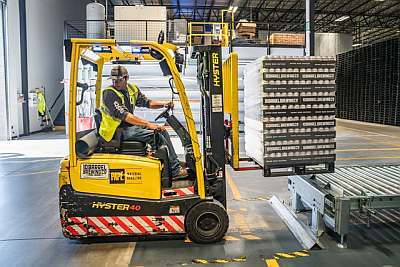# Inventory Turnover Calculator

Instructions: You can use our Inventory Turnover Calculator, by providing the Cost of Goods Sold $$(COGS)$$, the current inventory and the previous inventory in the form below:Cost of Goods Sold $$(COGS)$$ =Current Inventory =Previous Inventory =

## Inventory Turnover Calculator

More about the inventory turnover so you can better use the results provided by this solver.

The inventory turnover is the ratio between the cost of goods sold and the average inventory. This ratio is a measure of asset management, and it indicates the number many times a firm turns its inventory.

### How is inventory turnover calculated?

The inventory turnover is a financial ratio of efficiency, which is particularly useful when compared to that of other firms in the industry. It is computed using the following formula:

$\text{Inventory Turnover} = \displaystyle \frac{\text{Cost of Goods Sold}}{\text{Average Inventory}}$

Observe that, and this is a point that often brings confusion, the formula uses the average inventory, and just the last inventory.

### What is the ideal inventory turnover ratio for a company?

The Inventory Turnover Ratio is a commonly used financial ratio to measure efficiency in asset management. You can find other financial ratio calculators in our site, such as our current ratio , quick ratio , and our inventory turnover calculator.

### More finance calculators

Observe that the Cost of Goods Sold are also used to compute the Days' Sales in Inventory , another useful efficiency ratio.### What is an inventory turnover example?

Question: Assume that the cost of goods sold is $10,000, the current inventory is$1000 and the previous inventory is \$1300. Compute the inventory turnover based on these data.

Solution:

This is the information we have been provided with:

 Cost of Goods Sold $$COGS$$ = $$\text{\textdollar}10000$$ Current Inventory = $$\text{\textdollar}1000$$ Previous Inventory = $$\text{\textdollar}1300$$

Hence, the Inventory Turnover is computed using the following formula:

$\begin{array}{ccl} \text{Inventory Turnover} & = & \displaystyle \frac{\text{Cost of Goods Sold}}{\text{Average Inventory}} \\\\ \\\\ & = & \displaystyle \frac{\text{\textdollar}10000}{(\text{\textdollar}1000+\text{\textdollar}1300)/2} \\\\ \\\\ & = & \displaystyle \frac{\text{\textdollar}10000}{\text{\textdollar}1150} \\\\ \\\\ & = & 8.7 \end{array}$

Therefore, the inventory turnover is $$8.7$$, for the given cost of goods sold of $$COGS = \text{\textdollar}10000$$, current inventory of $$\text{\textdollar}1000$$, and previous inventory of $$\text{\textdollar}1300$$. This means that firm turns its inventory over $$8.7$$ times.